Question

# A 1.32 L buffer solution consists of 0.121 M butanoic acid and 0.345 M sodium butanoate....

A 1.32 L buffer solution consists of 0.121 M butanoic acid and 0.345 M sodium butanoate. Calculate the pH of the solution following the addition of 0.066 moles of NaOH. Assume that any contribution of the NaOH to the volume of the solution is negligible. The Ka of butanoic acid is 1.52 × 10-5.

A 1.44 L buffer solution consists of 0.326 M propanoic acid and 0.103 M sodium propanoate. Calculate the pH of the solution following the addition of 0.062 moles of HCl. Assume that any contribution of the HCl to the volume of the solution is negligible. The Ka of propanoic acid is 1.34 × 10-5.

A buffer with a pH of 4.38 contains 0.27 M of sodium benzoate and 0.18 M of benzoic acid. What is the concentration of [H ] in the solution after the addition of 0.054 mol of HCl to a final volume of 1.4 L? Assume that any contribution of HCl to the volume is negligible.

Buffer capacity is a measure of a buffer solution\'s resistance to changes in pH as strong acid or base is added. Suppose that you have 185 mL of a buffer that is 0.140 M in both propanoic acid (C2H5COOH) and its conjugate base (C2H5COO–). Calculate the maximum volume of 0.190 M HCl that can be added to the buffer before its buffering capacity is lost.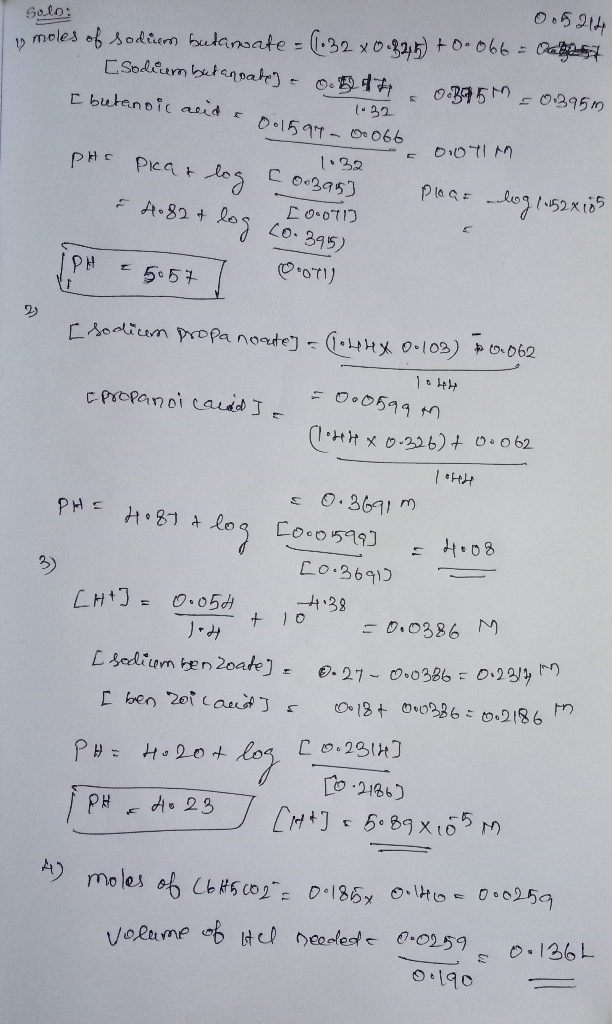#### Earn Coins

Coins can be redeemed for fabulous gifts.

Similar Homework Help Questions
• ### A 1.37 L buffer solution consists of 0.153 M butanoic acid and 0.326 M sodium butanoate....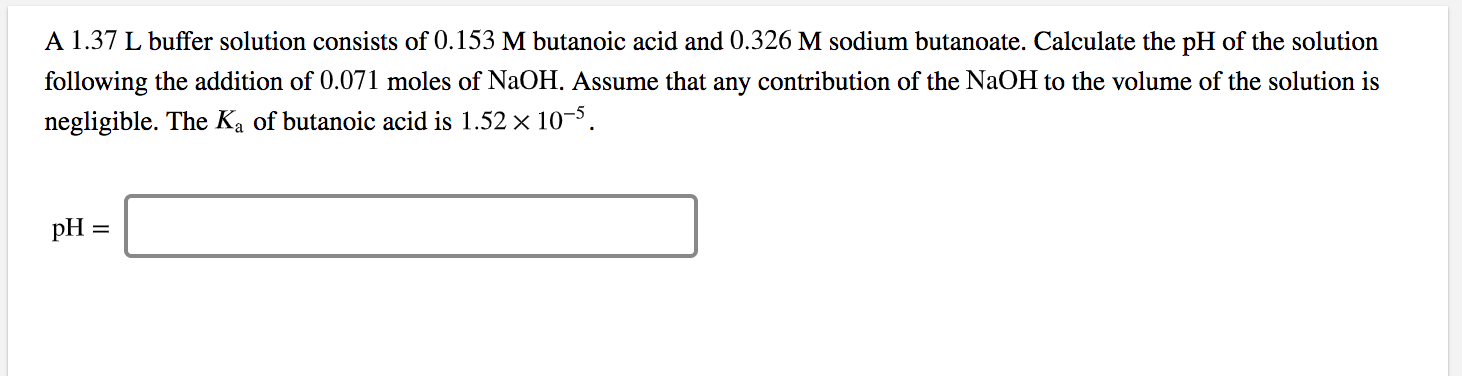A 1.37 L buffer solution consists of 0.153 M butanoic acid and 0.326 M sodium butanoate. Calculate the pH of the solution following the addition of 0.071 moles of NaOH. Assume that any contribution of the NaOH to the volume of the solution is negligible. The Ka of butanoic acid is 1.52 x 10-5. pH =

• ### A 1.40 L buffer solution consists of 0.313 M propanoic acid and 0.121 M sodium propanoate....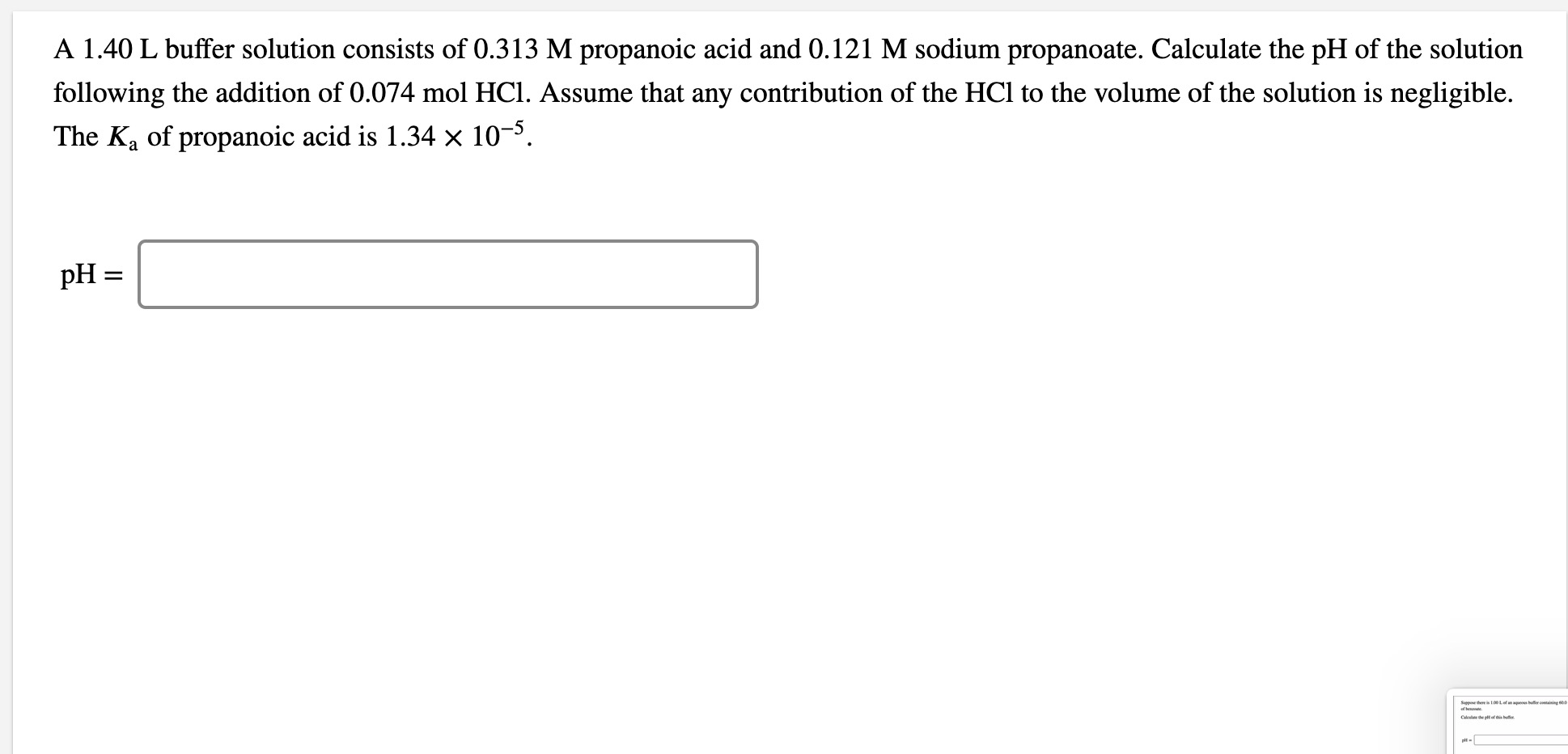A 1.40 L buffer solution consists of 0.313 M propanoic acid and 0.121 M sodium propanoate. Calculate the pH of the solution following the addition of 0.074 mol HCl. Assume that any contribution of the HCl to the volume of the solution is negligible. The Ka of propanoic acid is 1.34 x 10-5. pH = Cape

• ### A 1.36 L buffer solution consists of 0.157 M butanoic acid and 0.311 M sodium butanoate....

A 1.36 L buffer solution consists of 0.157 M butanoic acid and 0.311 M sodium butanoate. Calculate the pH of the solution following the addition of 0.070 moles of NaOH. Assume that any contribution of the NaOH to the volume of the solution is negligible. The ?a of butanoic acid is 1.52×10−5.

• ### A 1.41 L buffer solution consists of 0.154 M butanoic acid and 0.280 M sodium butanoate....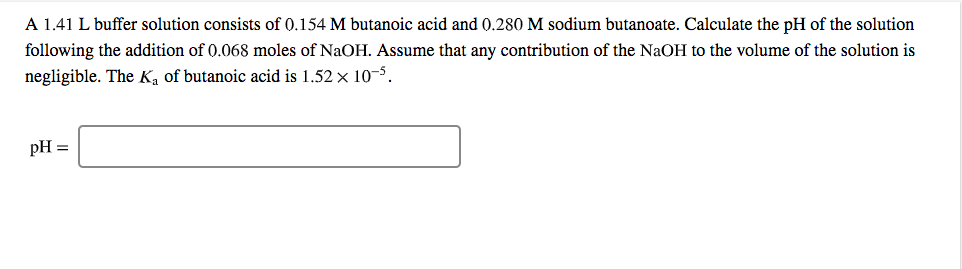A 1.41 L buffer solution consists of 0.154 M butanoic acid and 0.280 M sodium butanoate. Calculate the pH of the solution following the addition of 0.068 moles of NaOH. Assume that any contribution of the NaOH to the volume of the solution is negligible. The K, of butanoic acid is 1.52 x 10-5. pH =

• ### A 1.35 L buffer solution consists of 0.115 M butanoic acid and 0.331 M sodium butanoate....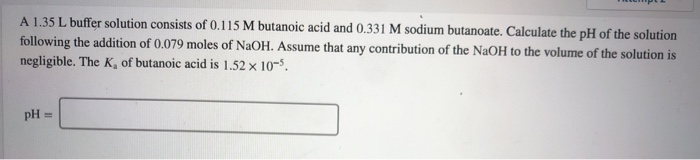A 1.35 L buffer solution consists of 0.115 M butanoic acid and 0.331 M sodium butanoate. Calculate the pH of the solution following the addition of 0.079 moles of NaOH. Assume that any contribution of the NaOH to the volume of the solution is negligible. The K, of butanoic acid is 1.52 x 10-5. pH =

• ### A 1.46 L buffer solution consists of 0.108 M butanoic acid and 0.303 M sodium butanoate....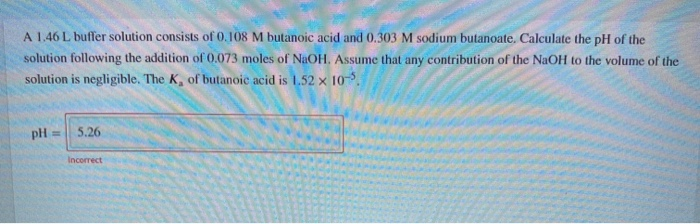A 1.46 L buffer solution consists of 0.108 M butanoic acid and 0.303 M sodium butanoate. Calculate the pH of the solution following the addition of 0.073 moles of NaOH. Assume that any contribution of the NaOH to the volume of the solution is negligible. The K, of butanoic acid is 1.52 x 10-5 pH = 5.26 Incorrect

• ### A 1.47 L buffer solution consists of 0.329 M propanoic acid and 0.188 M sodium propanoate....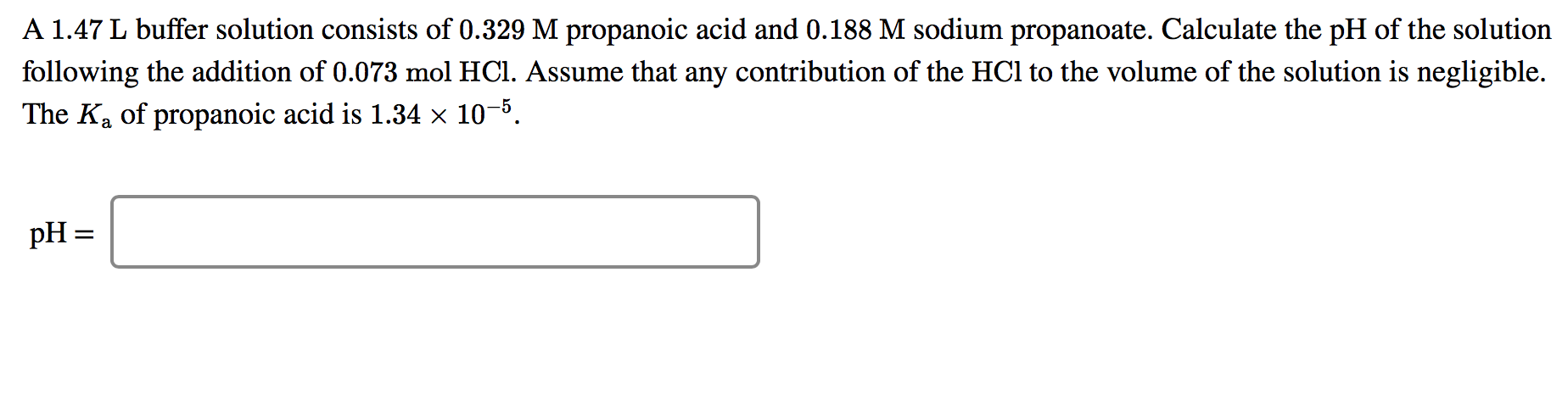A 1.47 L buffer solution consists of 0.329 M propanoic acid and 0.188 M sodium propanoate. Calculate the pH of the solution following the addition of 0.073 mol HCl. Assume that any contribution of the HCl to the volume of the solution is negligible. The Ka of propanoic acid is 1.34 x 10-5. pH=

• ### A 1.33 L buffer solution consists of 0.274 M propanoic acid and 0.200 M sodium propanoate....

A 1.33 L buffer solution consists of 0.274 M propanoic acid and 0.200 M sodium propanoate. Calculate the pH of the solution following the addition of 0.065 mol HCl. Assume that any contribution of the HCl to the volume of the solution is negligible. The Ka of propanoic acid is 1.34×10−5

• ### A 1.37 L buffer solution consists of 0.251 M propanoic acid and 0.130 M sodium propanoate....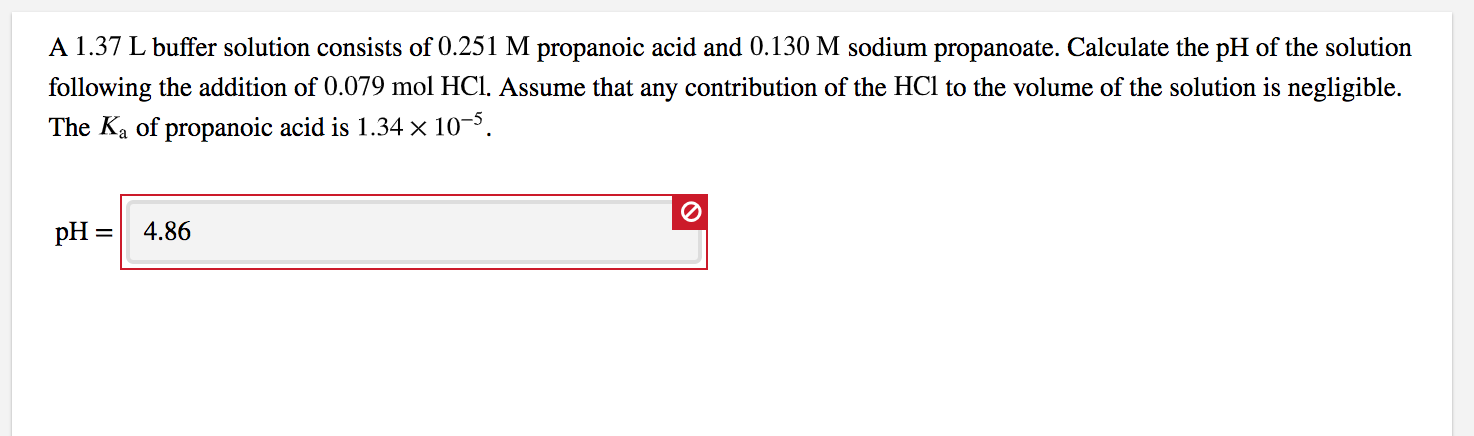A 1.37 L buffer solution consists of 0.251 M propanoic acid and 0.130 M sodium propanoate. Calculate the pH of the solution following the addition of 0.079 mol HCl. Assume that any contribution of the HCl to the volume of the solution is negligible. The Ka of propanoic acid is 1.34 x 10-5. pH = 4.86

• ### A 1.421.42 L buffer solution consists of 0.1230.123 M butanoic acid and 0.3050.305 M sodium butanoate....

A 1.421.42 L buffer solution consists of 0.1230.123 M butanoic acid and 0.3050.305 M sodium butanoate. Calculate the pH of the solution following the addition of 0.0630.063 moles of NaOHNaOH. Assume that any contribution of the NaOHNaOH to the volume of the solution is negligible. The ?aKa of butanoic acid is 1.52×10−51.52×10−5.